Courses
Courses for Kids
Free study material
Free LIVE classes
More

# LC Oscillations - Mathematical Formulation with Working for JEELIVE
Join Vedantu’s FREE Mastercalss

## What is LC Oscillator?

An LC oscillation is a circuit that is composed of the capacitor and inductor. In this circuit, the capacitor is fully charged and linked to the uncharged inductor. In LC oscillation, an electric current is set up and undergoes the LC oscillations when a charged capacitor is linked with the inductor. An LC oscillator is a type of circuit comprising  a capacitor and inductor which is designed in such a way that the required oscillations of positive feedback will continue working in the circuit. An oscillator circuit is a type of circuit that is used to convert the DC signal into an AC signal in the form of waves.

In this article, you’’ understand about the concept of LC oscillations and LC oscillator circuits. We will also discuss the types of LC oscillators, frequency of LC oscillator, LC Oscillator derivation, time period of LC oscillation, and working of LC oscillator.

## LC Oscillator Working

This section discusses the working of the LC oscillator in an elaborate manner. The following stages have been mentioned for the understanding of the working of the LC Oscillator:

• This is the beginning stage of the working of the LC Oscillator. In this stage, a fully powered capacitor is connected with the uncharged inductor. Due to this, the Capacitor captures all the energy of the circuit and the inductor remains de-energized. A circuit diagram has been explained for a better understanding of the first stage. The energy stored in the capacitor is depicted by UE and the energy stored in the inductor is depicted by UB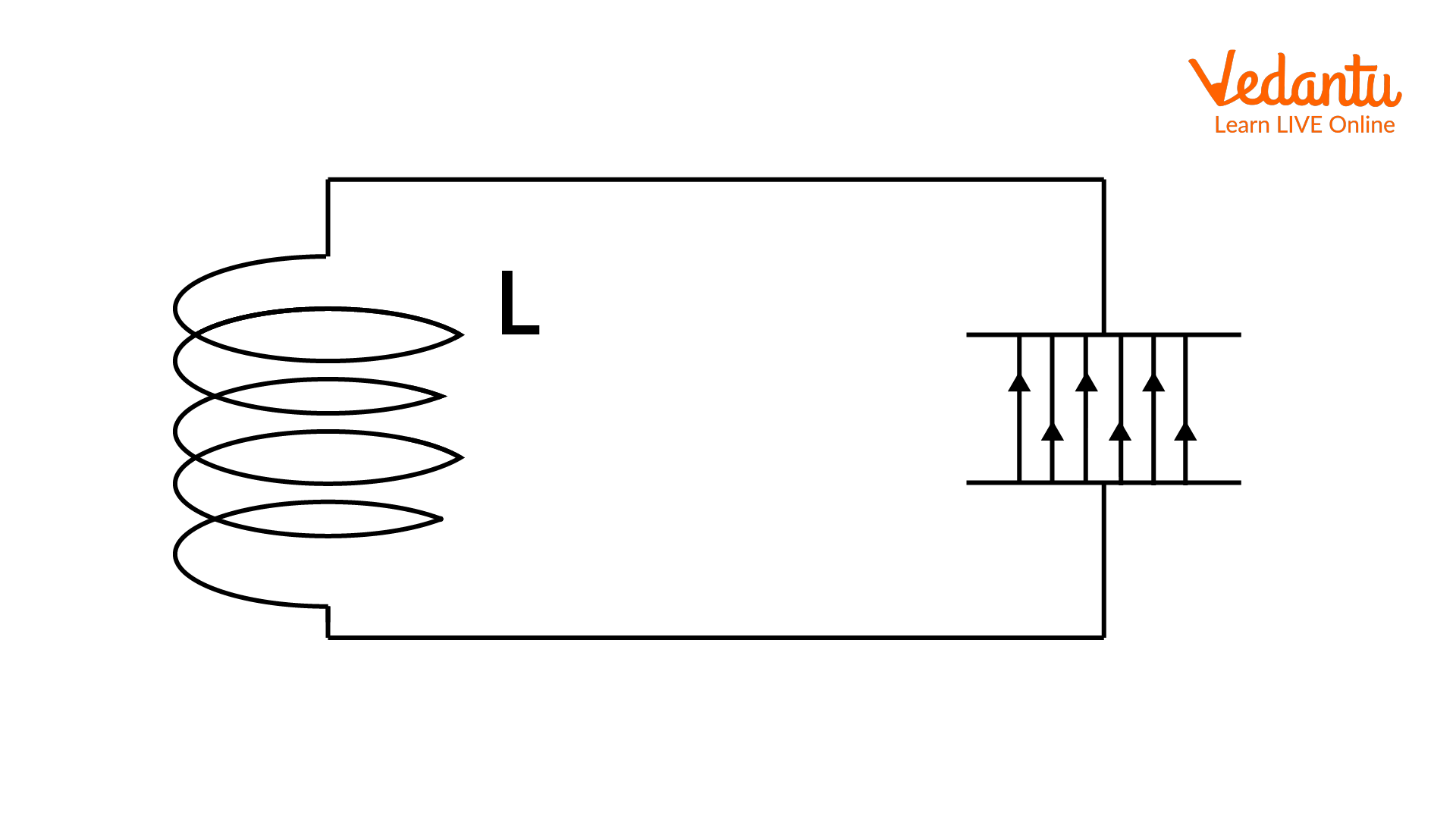A LC Oscillator Circuit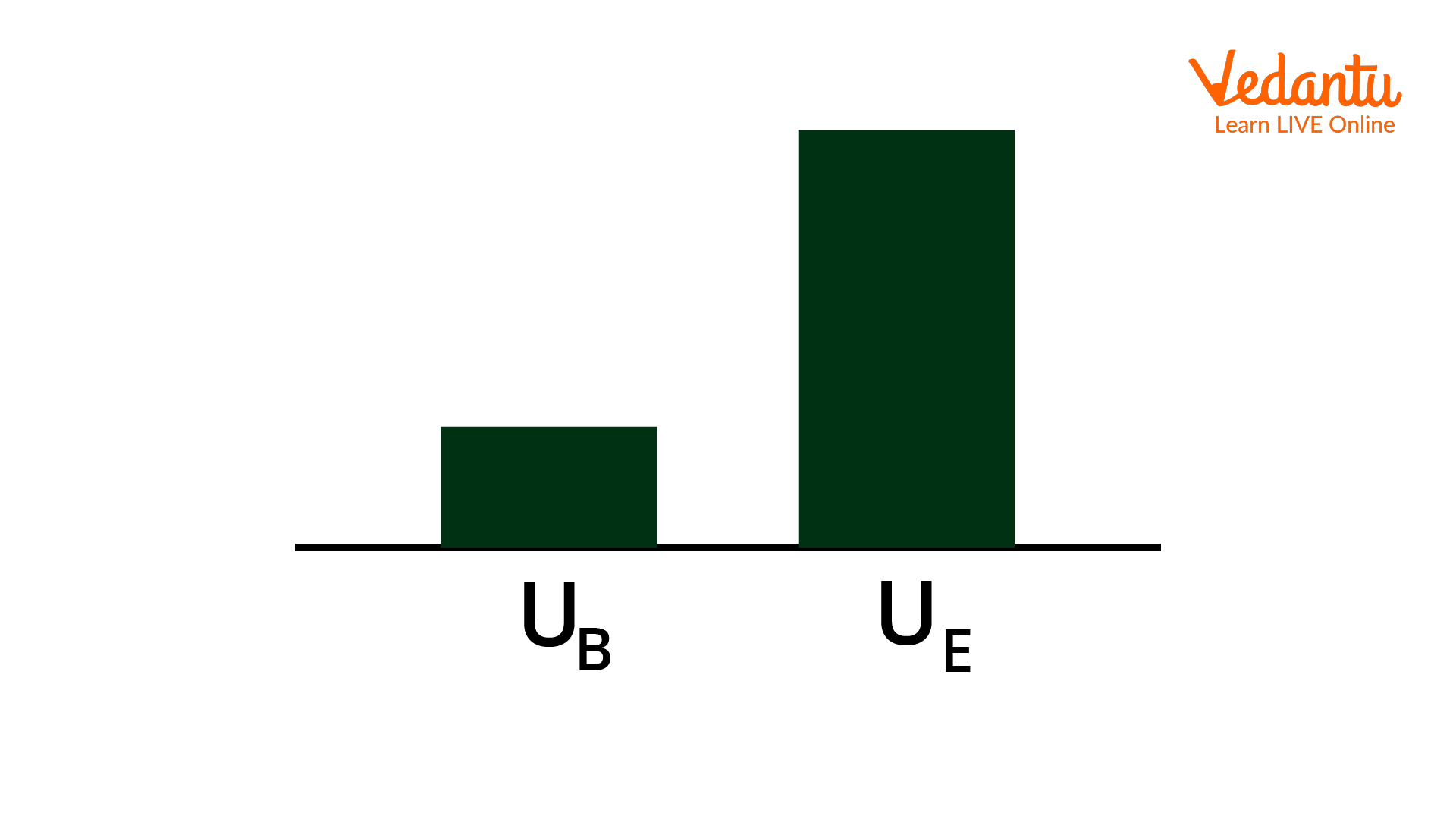Depiction of Graphs of Energy Storage

• In stage 2, the capacitor starts energizing the inductor by flowing the electric current to the inductor. The graphs clearly explain that the capacitor starts falling in energy level while the energy of the inductor begins to rise. A circuit diagram along with the graph depicts the current of the inductor and capacitor.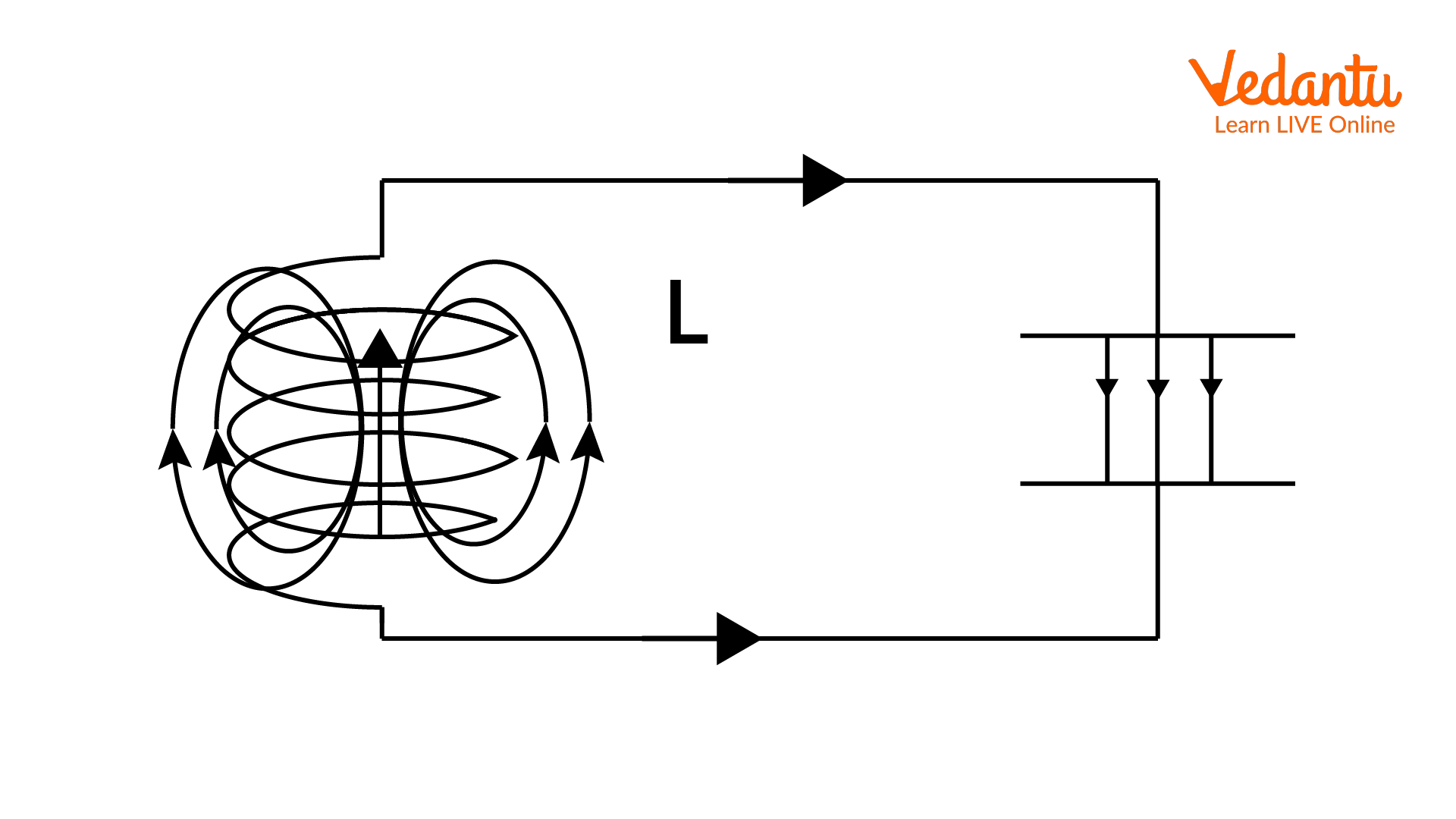A LC Oscillator Circuit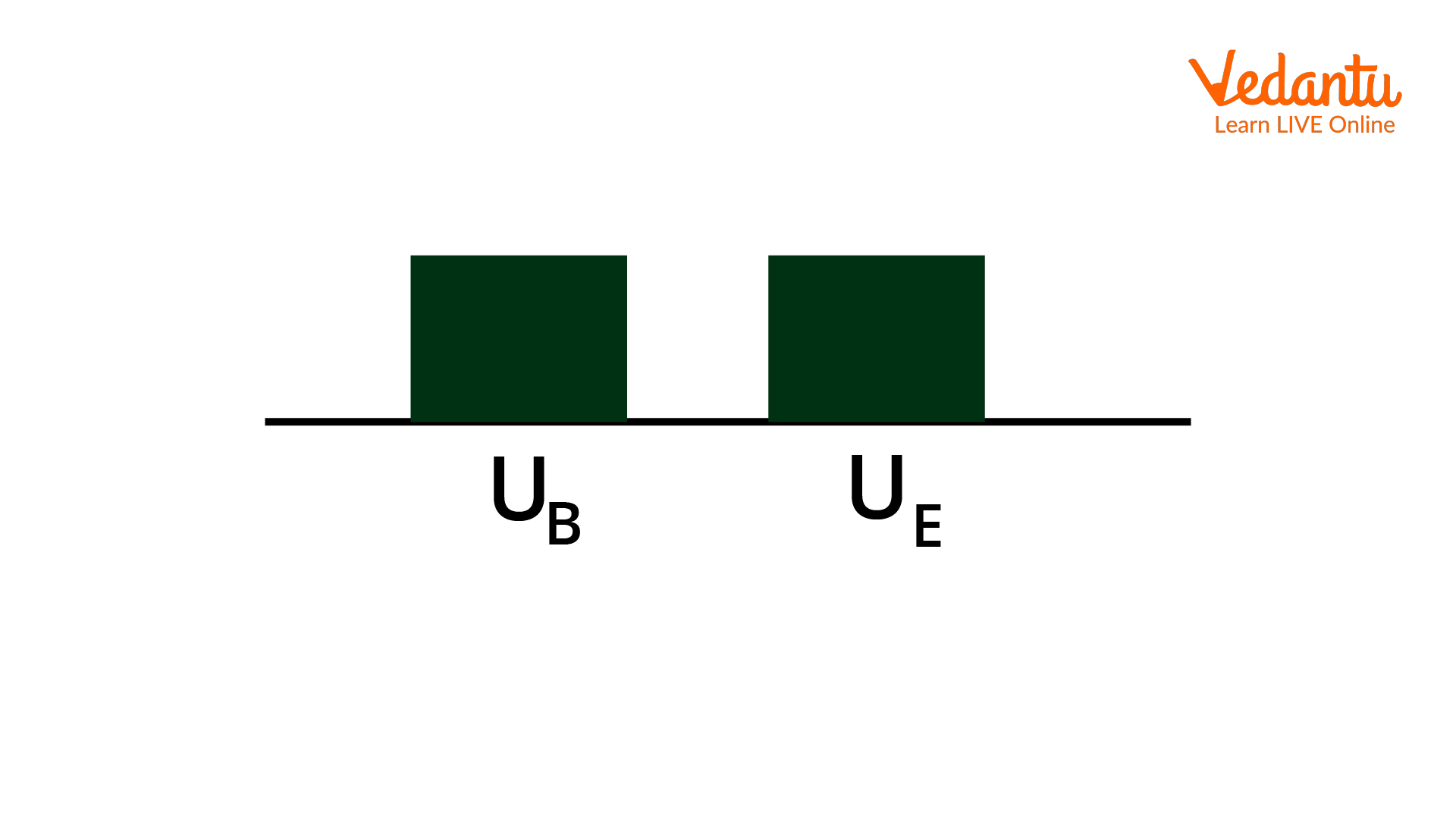Depiction of Graphs of Energy Storage

• In Stage 3, the energy stored in the capacitor is transferred by half amount to the inductor by flowing current through it. The energy storage graph depicts the transfer of half amount of energy from the capacitor to the inductor. The graph has shown an equivalent size of the bar graph depicting the half amount of transfer of energy from the capacitor to the inductor.A LC Oscillator CircuitDepiction of Graphs of Energy Storage

• In Stage 4, the entire amount of energy that was stored in the capacitor was transferred to the inductor. As the amount of energy that was drained from the capacitor, the transmitted energy to the inductor will be transformed into the magnetic energy. The image of the given circuit diagram will depict the current state of the working LC oscillator.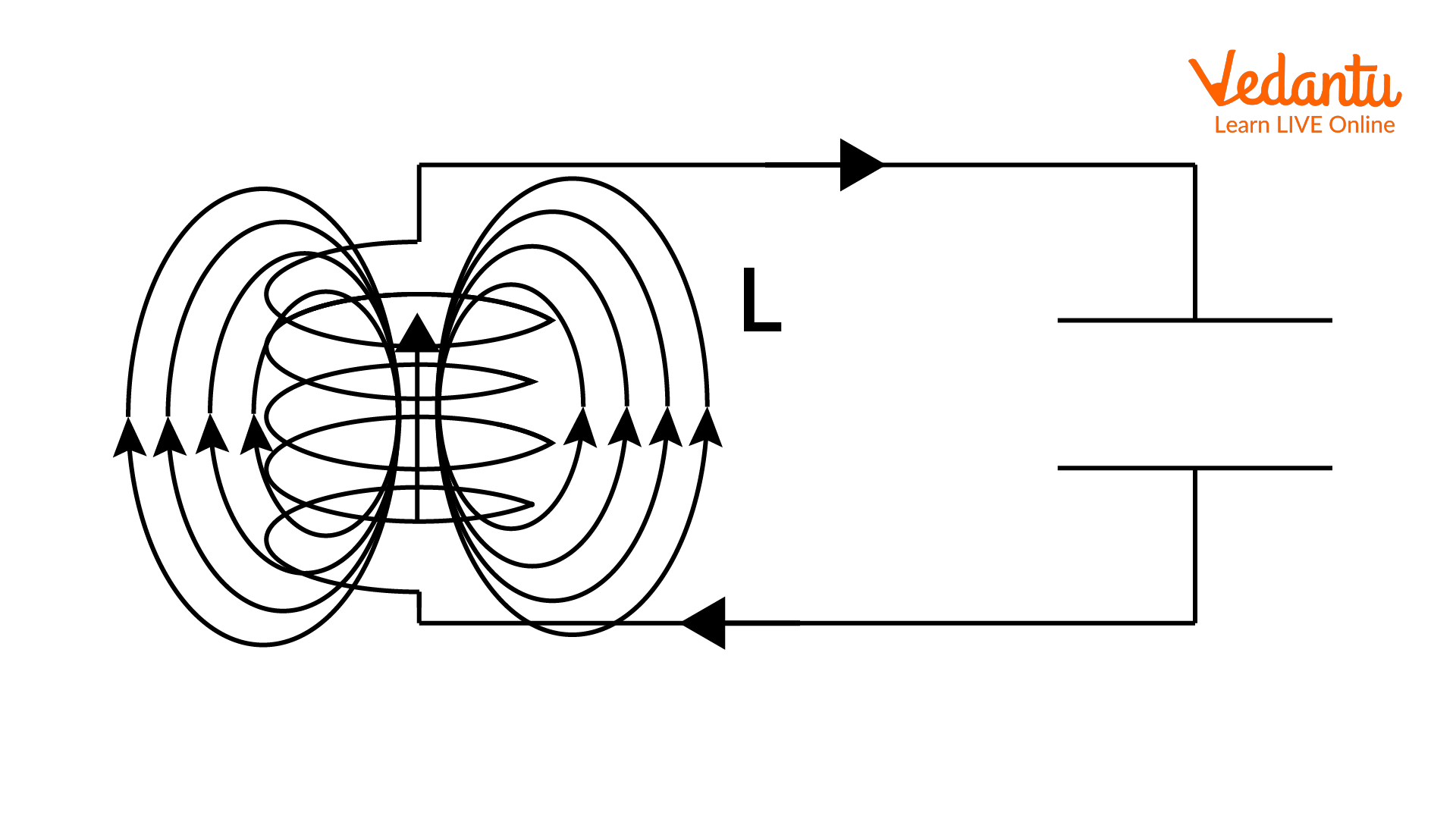A LC Oscillator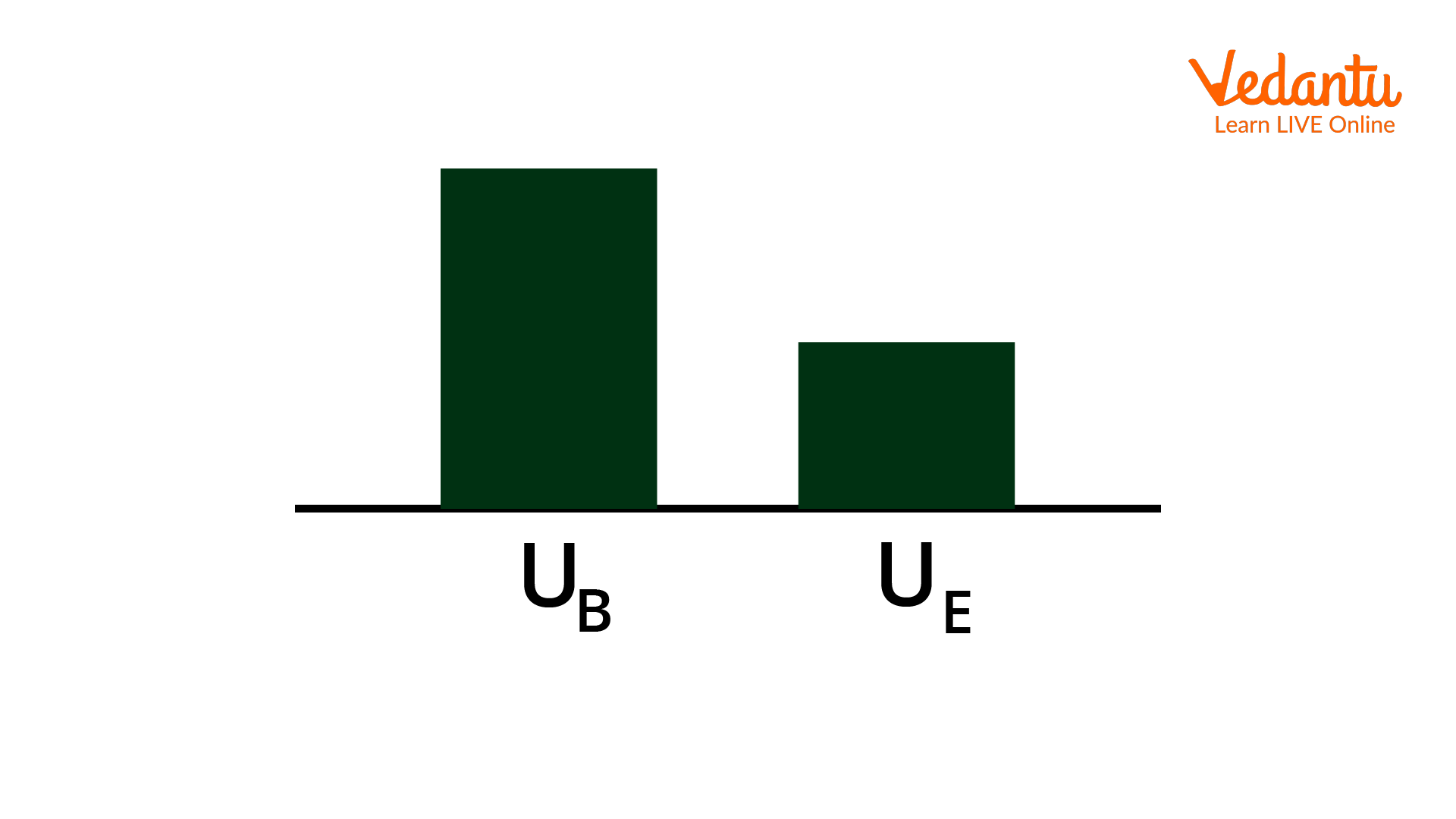Depiction of Graphs of Energy Storage

• In stage five, the energy of the capacitor is found to be completely drained and the transferred energy from the capacitor to the inductor makes the inductor fully energized. Now, the inductor will start to charge the capacitor in the same direction as the current.  The current states will be depicted in the following circuit diagram and graphs of the energy storage of the capacitor and inductor.A LC OscillatorDepiction of Graphs of Energy Storage

• The sixth stage of the working of the LC Oscillator is the final stage of the working process. In this stage, the energy of the capacitor will become full and it again works as a charged capacitor. On the other hand, the energy of the inductor will again be de-energized. Now, when the current starts flowing in the circuit, the current flowing direction will be opposite to the initial condition.A LC Oscillator Circuit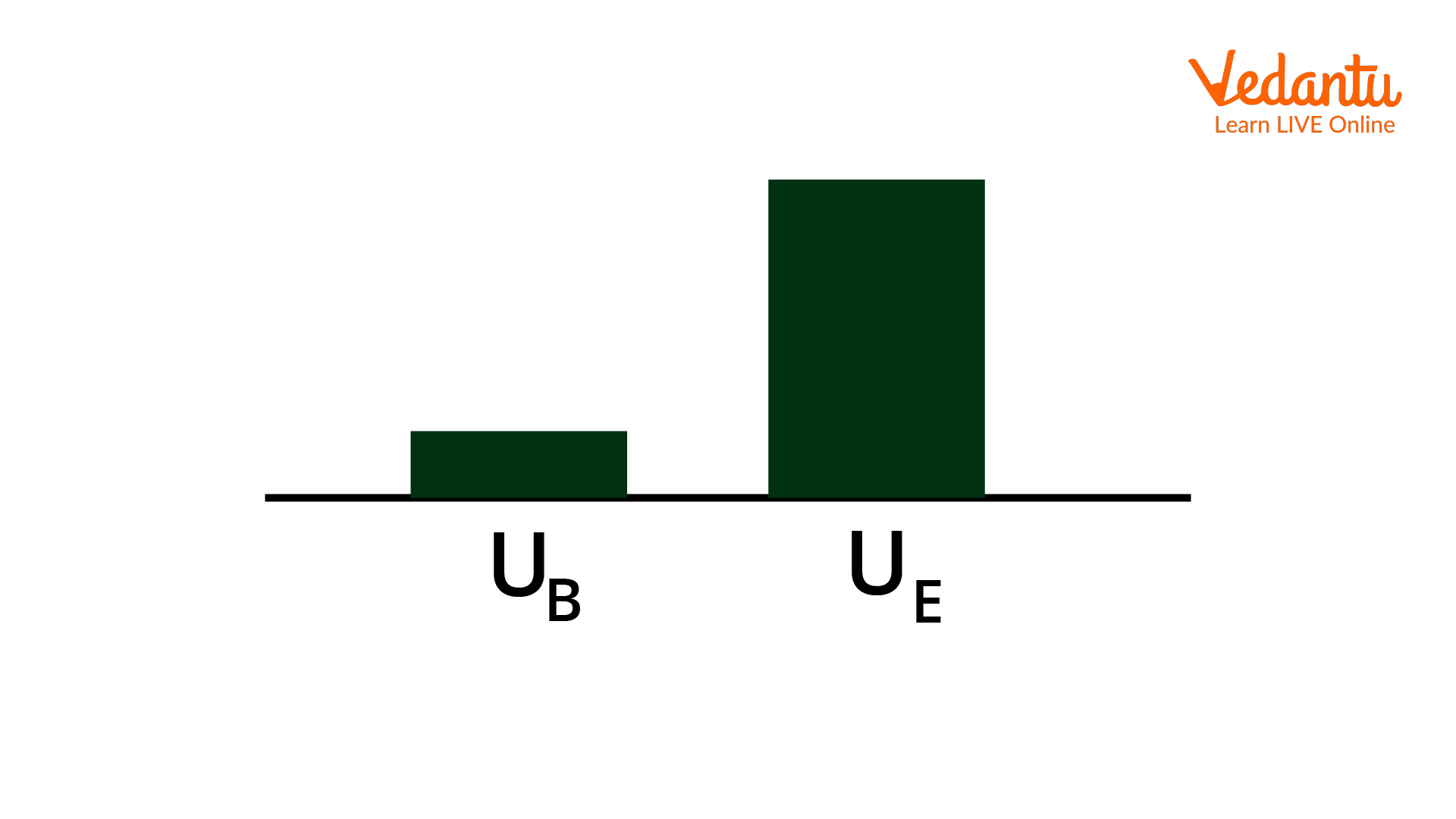Depiction of Graphs of Energy Storage

In conclusion, both the major components of this circuit will again charge twice in the complete cycle of the process of the LC Oscillator.

## A Mathematical Formulation of LC Oscillation

This section derives the LC oscillation formula, derivation of the time period of LC Oscillation, and frequency of LC oscillation. For determination of the frequency and time period of the LC, it is necessary to determine the differential equation of LC oscillation,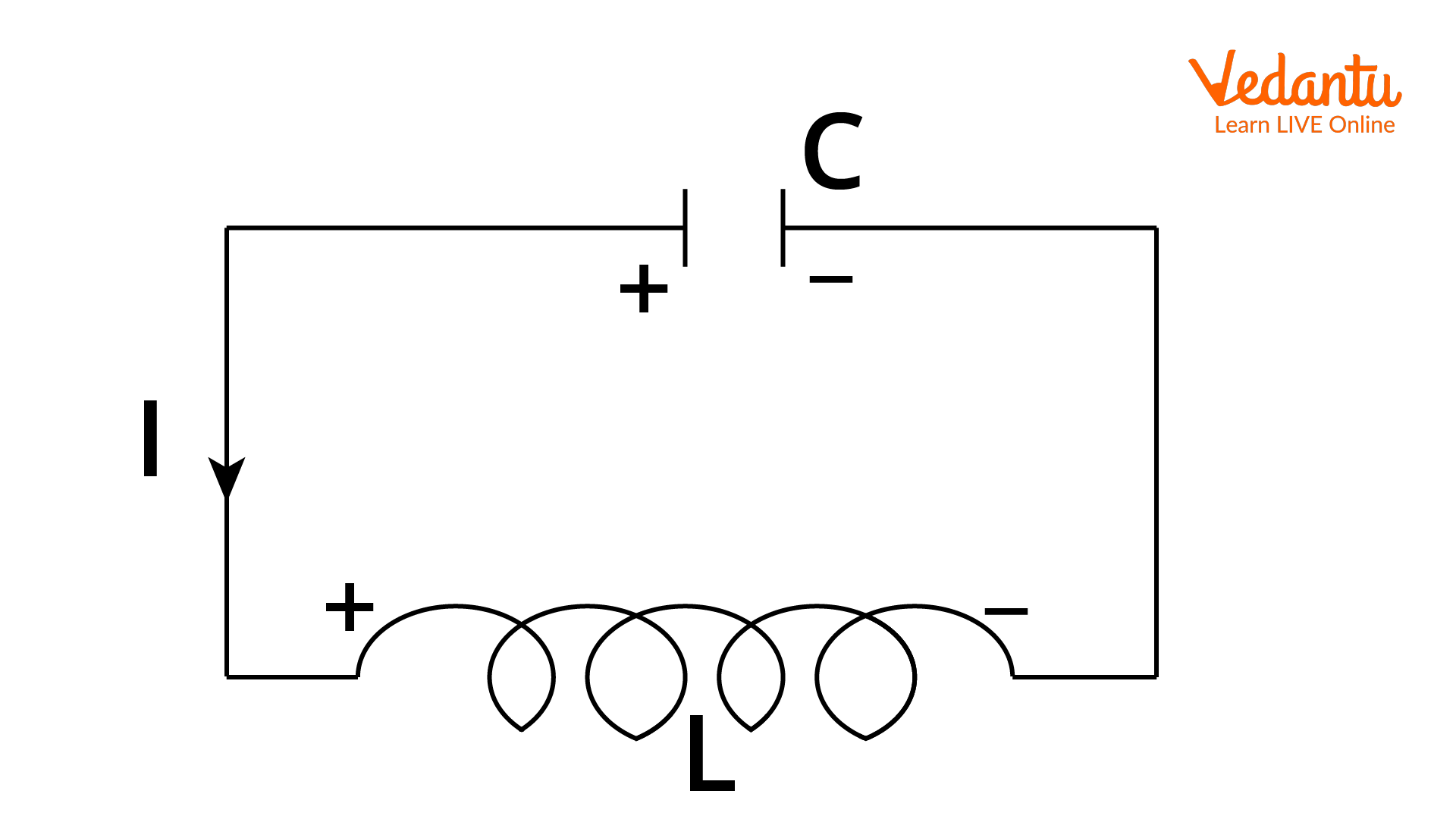A Schematic Diagram of The LC Oscillator

When Kirchhoff's Voltage law is applied to the circuit, the magnitude of the total potential difference across the capacitor and inductor will be zero. Therefore, it means that

\begin{align} &V_{L}+V_{C}=0 \\ &-L \dfrac{d i}{d t}+\dfrac{q}{C}=0 \end{align}

It is known that the current is the flow of charge per unit, it is written as

$i=\dfrac{-dq}{dt}$

As the amount of current will become minimum when current starts flowing from the capacitor to the inductor, substitute the value of current in the above equation. Hence,

\begin{align} &-L \dfrac{d}{d t}\left(\dfrac{-d q}{d t}\right)+\dfrac{q}{C}=0 \\ &L \dfrac{d^{2} q}{d t^{2}}+\dfrac{q}{C}=0 \\ &\dfrac{d^{2} q}{d t^{2}}=-\dfrac{q}{L C} \\ &\dfrac{d^{2} q}{d t^{2}}+\dfrac{q}{L C}=0 \end{align}

This differential equation for LC oscillations is given by the above equation. Therefore, the angular frequency of LC Oscillations will be written as:

$\dfrac{d^{2} q}{d t^{2}}+q \omega^{2}=0$

On comparing the angular frequency will be given as

$\omega=\dfrac{1}{\sqrt{LC}}$

It is known that $\omega=2\pi f$. Therefore,

$f=\dfrac{1}{2\pi\sqrt{LC}}$

Hence, the time period for LC oscillation will be derived,

\begin{align} &T=\dfrac{1}{f} \\ &T=\dfrac{1}{\dfrac{1}{2 \pi \sqrt{L C}}} \\ &T=2 \pi \sqrt{L C} \end{align}

Therefore, the time period of LC oscillation is obtained $2\pi\sqrt{LC}$ and the frequency of LC oscillation is obtained $\dfrac{1}{2\pi\sqrt{LC}}$.

## Types of LC Oscillator

There are five types of LC oscillators which are as shown below:

1. Tuned Collector Oscillator

This is one of the basic types of LC oscillators. This circuit can be easily formed with the capacitor and the transformer which are connected in parallel across the oscillator’s Collector circuit. The tank in this circuit is formed by a transformer and capacitor. The oscillations will be generated by the transformer’s feed within the circuit to the base of the transistors.

1. Tuned Base Oscillator

One of the kinds of LC transistor oscillators in which the location of the circuit lies in between the two terminals of the transistors, i.e ground and the base. This circuit is formed with the help of a capacitor and the main coil of the transformer.

1. Hartley Oscillator

This is a different type of LC oscillator in which the tank circuit includes two inductors and one capacitor. The connections are made in such a way that the capacitor is connected in parallel and the inductors are connected in series and the combination of both is also connected in series. It is known as the Hartley oscillator because it was invented by Ralph Hartley in 1915.

1. Colpitts Oscillator

One type of LC oscillator is the Colpitts oscillator in which the tank circuit is built in such a way that one inductor and two capacitors are used in it. The connection of this circuit is connected in a way where the connections between capacitors are done in series with the parallel connection to the inductor.

1. Clapp Oscillator

This LC Oscillator is a modification of the Colpitts oscillator. In this oscillator, an extra capacitor is connected in the series with the inductor within the tank circuit.  The extra capacitor tries to separate the other two capacitors from the junction of a transistor for advances the frequency

## Conclusion

We have brief information regarding the concept of LC Oscillation and LC oscillator along with the circuit diagram. We also discussed the derivation of the frequency and time period of the LC oscillation, and also classified the types of LC oscillators and explained the working of the LC Oscillator. The article starts with the clarification of the first query, i.e. what is LC Oscillation. This article explained the different types of oscillators along with the connection of the capacitor with the inductor and transistor. It covers the concept of LC Oscillator from a diagram point of view for better clarity of concept.

Last updated date: 23rd Sep 2023
Total views: 135.9k
Views today: 3.35k

## FAQs on LC Oscillations - Mathematical Formulation with Working for JEE

1. What is the principle of LC Oscillator?

The principle behind the working of the LC oscillator is to use a tank circuit that comprises the capacitor and inductor. In this circuit, whenever the voltage is applied to the circuit, the capacitor of the circuit gets charged. When the battery shuts down, the charging of the capacitor will stop and the stored energy of the capacitor will be used for charging the inductor. When the capacitor gets discharged, the inductor will charge the capacitor in the same way.

2. What is the application area of the LC Oscillator?

The application of the LC Oscillator is distributed in various electrical sectors. These oscillators are broadly applied for the generation of high-frequency signals. Those oscillators which are used for generating such high-frequency signals are known as RF oscillators. By following the practical values of the capacitors and inductors. The maximum range of generated frequency is more than 500MHz. The most applications of the LC oscillators are found in television, radio, RF generators, etc. hence, these are the application areas of the LC Oscillators.

3. What is the weightage of this topic in the JEE exam?

The weightage of the current topic titled LC oscillator is 3%. The chapter on oscillation and waves was found to be an important topic for the JEE. Nearly 2-3 questions can be expected in the given competitive examination of JEE. In this chapter, it is required to keep a check on every topic and practice a maximum number of questions for better performance in this section. Hence, it is required to give importance to this chapter from JEE's point of view.